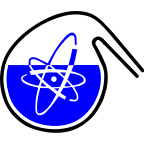#RadChem 2018

13-18 May 2018
Casino Conference Centre
Europe/Prague timezone

## A comparative study of radium and strontium uptake by cementitious materials

17 May 2018, 17:30
1h 30m
Gallery (Casino Conference Centre)

### Gallery

#### Casino Conference Centre

Reitenbergerova 4/95, Mariánské Lázně, Czech Republic
Poster Separation Methods, Speciation

Jana Kittnerová

### Description

Cementitious materials will be very important structural elements of a deep geological repository, but they can be used also as a part of engineering barriers. A$~$comparative study of radium and strontium uptake by different cementitious materials was therefore performed. Three cementitious materials were studied: a$~$hardened cement paste (HCP CEM II) and two types of concrete (Concrete CEM I and Concrete CEM III, both actually utilized in the storages of institutional radioactive waste in Czech Republic); all crushed and sieved on fraction ≤$~$0.4 mm. The$~$cementitious materials were analysed by X-ray diffraction and thermogravimetric analysis and used to the comparative study of equilibrium sorption of the $^{223}$Ra (used as an analogue of $^{226}$Ra) and Sr with radiotracer $^{85}$Sr under various conditions. On$~$same materials, the diffusion experiments with both isotopes were also done.
Sorption experiments with $^{223}$Ra were carried out in a carrier-free arrangement, concentration of $^{223}$Ra was approximately 1·10$^{-12}$$~mol·L^{-1}. Sorption experiments with Sr were carried out mainly with concentration of Sr c~=~3.5∙10^{-4}$$~$mol·L$^{-1}$ traced with $^{85}$Sr, but some of the experiments were also done as carrier free. However, all the cementitious materials used contain Sr, which may affect the experiment. Varying conditions included the temperature, the liquid-to-solid ratio V/m, and the composition of the starting solution which was either Portlandite water (saturated Ca(OH)$_2$) or synthetic water CW based on granitic water equilibrated with Ca(OH)$_2$, which is a$~$representative of cement water.
Comparing obtained K$_d$ values corresponding to Sr and Ra uptake, it was determined that Sr reaches distribution coefficients in range of 10$~$–$~$150$~$L·kg$^{-1}$ and Ra in 70$~$–$~$900$~$L·kg$^{-1}$. The$~$influence of rising temperature (over 50 °C) on the sorption of both elements was observed – rising temperature increases K$_d$ values, especially for lower liquid-to-solid ratio. In the case of Ra, higher K$_d$ when the liquid phase was changed from Portlandite water to CW were reached, but there is no significant influence of the liquid phase change for Sr. Liquid-to-solid ratio also affects differently results of the experiments. In the range of V/m from 10 to 600$~$L·kg$^{-1}$, K$_d$ values are mostly comparable for Sr, the K$_d$ for Ra are rising in general. The$~$steep increase of K$_d$ for V/m$~$=$~$1000$~$L·kg$^{-1}$ for both elements was observed, accompanied by unclear influence of rising temperature for this phase ratio too.

### Co-authors

Barbora Drtinova (CTU Prague) Dušan Vopálka (CTU in Prague, FNSPE, katedra jaderné chemie)

### Presentation Materials

There are no materials yet.